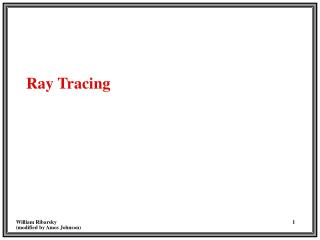DownloadDownload PresentationRay Tracing

# Ray Tracing

Télécharger la présentation## Ray Tracing

- - - - - - - - - - - - - - - - - - - - - - - - - - - E N D - - - - - - - - - - - - - - - - - - - - - - - - - - -
##### Presentation Transcript

1. Ray Tracing

2. Trace light rays that enter eye through view-plane back from whence they came. (# rays == # pixels) Use illumination model to determine color at intersection of ray and object. Shadows “happen”. Ray Tracing Light Source Shadow Ray Center of Projection Projection Plane

3. BASIC RAY TRACING 1. Find intersection of ray with nearest object in scene (Eye ray). 2. Determine if that object is in shadow relative to light source(s). (Shadow ray(s)). 3. Determine illumination of object from light source(s). A LARGE part of the work is in calculating intersections. Performance can be improved by making intersection calculations more efficient or by avoiding unnecessary intersection calculations.

4. Ray is defined by R(t) = R0 + Rd*t where t > 0 R0 = Origin of ray at (x0, y0, z0) Rd = Direction of ray [xd, yd, zd] unit vector Plane is defined by [A, B, C, D] Ax + By + Cz + D = 0 for a point in the plane A2 + B2 + C2 = 1 Normal Vector, N = [A, B, C] Ray/Plane Intersection

5. Substitute the ray equation into the plane equation: A(x0 + xd*t ) + B(y0 + yd*t) + C(z0 +zd*t) + D = 0 Solve for t: t = - (Ax0 + By0 + Cz0 + D) / ( Axd + Byd + Czd) or t = - ( N• R0 + D) / ( N• Rd) Note: The normal vector of the plane should usually (except for backface removal) be for the surface facing the ray. If it isn't then you should reverse it. If N• Rd > 0 Then use -[A, B, C] Ray/Plane (cont.)

6. If N·Rd = 0, then the ray and plane are parallel and don’t intersect To Determine if the intersection point lies in the polygon Project the 3D plane onto a 2D surface, by remove the dimension with the largest absolute value in the plane equation Use the “inside/outside” test for 2D polygons to determine if the point lies inside or outside. Ray/Plane (cont.)

7. Ray is defined by R(t) = R0 + Rd*t where t > 0 R0 = Origin of ray at (x0, y0, z0) Rd = Direction of ray [xd, yd, zd] unit vector A sphere with center (a, b, c) and radius r may be represented by (x - a)2 + (y - b)2 + (z - c)2 - r2 = 0 Ray/Sphere Intersection

8. Substitute the basic ray equation x = x0 + xd*t y = y0 + yd*t z = z0+ zd*t into the equation of the sphere (x0 + xd*t - a)2 + (y0 + yd*t - b)2 + (z0 +zd*t - c)2 - r2 = 0 This is a quadratic equation in t! A*t 2 + B*t + C = 0 where A = (xd)2 + (yd)2 + (zd)2 B = 2[xd (x0 - a) + yd (y0 - b) + zd ( z0 - c)] C = (x0 - a)2 + (y0 - b)2 + (z0 - c)2 - r2 Solving for t

9. If there are no real roots, then the ray and sphere do not intersect If there is only one real root, then the ray grazes the sphere If there are two real roots, then the ray intersects the sphere The root that gives the smallest positive t is the closet intersection point Solving for t (cont.) 1. 3. 2.